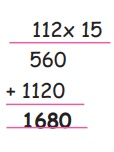Home | | Maths 5th Std | Multiply the three digit numbers by two digit numbers

# Multiply the three digit numbers by two digit numbers

Multiply the three digit numbers by two digit numbers

Multiply the three digit numbers by two digit numbers

Example 1

Raveena has planted 15 rows of coconut trees in her garden. Each row has 112 trees. How many coconut trees were planyed in total?

Using multiply method

Number of rows planted by Raveena = 15

Number of trees in one row = 112

Total number of coconut trees in her garden = 112×15

= 1680Example 2

One kg of Apple is sold by Bathri for Rs 165. Find the total cost of 12 Kgs of Apple?

Cost of 1Kg of Apple = Rs 165.

Total cost of 12 Kgs of Apple = 165 × 12

= Rs 1980Tags : Numbers | Term 1 Chapter 2 | 5th Maths , 5th Maths : Term 1 Unit 2 : Numbers
Study Material, Lecturing Notes, Assignment, Reference, Wiki description explanation, brief detail
5th Maths : Term 1 Unit 2 : Numbers : Multiply the three digit numbers by two digit numbers | Numbers | Term 1 Chapter 2 | 5th Maths